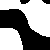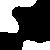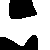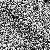### Justin's Graphical Models / Conditional Random Field Toolbox

This is a Matlab/C++ "toolbox" of code for learning and inference with graphical models. It is focused on parameter learning using marginalization in the high-treewidth setting. Though the code is, in principle, domain independent, I've developed it with vision problems in mind, particularly for learning Conditional Random Fields (CRFs). This means that the code is A) efficient (all the inference algorithms are implemented in C++) and B) can handle arbitrary graph structures.

There are, at present, a bunch of limitations:

• All the inference algorithms are for marginal inference. No MAP inference, at all.
• The code handles pairwise graphs only
• All variables must have the same number of possible values.
• For tree-reweighted belief propagation, a single edge appearance probability must be used for all edges
For vision, these are usually no big deal. In other domains, though, these might be showstoppers.

Size: (non-comment lines of code)

• Matlab: 4204
• C++: 1322

The code can be downloaded here. Note that it includes a set of binaries for various things for efficiency. I've included binaries for 64-bit OS X, 64-bit linux, and 64-bit Windows. (Many thanks to David Klein and Alexei Skurikhin for providing Windows Binaries!) If you compile the code for a different platform, please email me the binaries so they could be included for others.

This code is under the permissive MIT license.

All versions

• Version 1 (Nov 7 2011): JGMT1.zip (Obsolete) Initial Version.
• Version 2 (Jan 4 2012): JGMT2.zip (Obsolete) Bugfixes, binaries for linux, new generic train_crf inferface, multithreaded back-TRW switch from Eigen 2 to Eigen 3.
• Version 3 (Sep 9 2013): JGMT3.zip (Obsolete) More windows binaries, small bugfix for HoG features
• Version 4 (April 20 2015): JGMT4.zip minor bugfix to adapt to changes in external libraries.

Windows Binaries

• If you are interested in using the code on Windows, Alexei Skurikhin has provided a set of binaries. Most of these are provided with the main code above, however, there are also special binaries to use openMP multithreading, and some details on how to compile if the binaries do not work on your system.

Paper:

Common Problems (Last updated May 5, 2017):

• Many loss functions return the marginals that were computed to arrive at the loss. However, the pseudolikelihood (the point of which is that inference is not necessary) does not do this.
• The code has a particular convention for unlabled variables. Specifically, this toolbox uses a label of 0 to represent unlabelled variables. Note that this is not the same thing as a "background" label. If you have fully-labeled binary data, you should use labels of 1 and 2, and reserve 0 only for missing data. If you mistakenly use labels of 0 and 1, the model will happily learn to predict "1" everywhere.

Examples

The code can be used in a bunch of different ways, depending on if you are looking for a specific tool to use, or a large framework.

• Just use the low-level Inference algorithms, namely:
• Tree-Reweighted Belief Propagation + variants (Loopy BP, TRW-S)
• Mean-field
Take care of everything else yourself.
• Use the Differentiation methods (back-TRW or implicit differentiation) to calculate parameter gradients by providing your own loss functions. Do everything else on your own.
• Use the Loss methods (E.M., implicit_loss) to calculate parameter gradients by providing a true vector x and a loss name (univariate likelihood, clique likelihood, etc.) Unlike the above usages, these methods explicitly consider the conditional learning setting where one has an input and an output.
• Use the CRF methods to do almost everything (deal with parameter ties for a specific type of model, etc.) These methods consider specific classes of CRFs and given and input, output, loss function, inference method, etc. give the parameter gradient. Employing this gradient in a learning framework is straightforward.

### Example: A simple CRF for binary denoising

First, set up the parameters of the problem
```N     = 5;  % number of training images
siz   = 50; % size of training images
rho   = .5; % TRW edge appearance probability
nvals = 2;  % this problem is binary
```
Next, generate the graph for this CRF. (A simple pairwise grid)
```model = gridmodel(siz,siz,nvals);
```
Now, generate the data. Basically, we make noisy images, then smooth them to make the true (discrete) output values, and then add noise to make the input.
```for n=1:N
x{n} = round(imfilter(rand(siz),fspecial('gaussian',50,7),'same','symmetric'));
t = rand(size(x{n}));
noiselevel = 1.25;
y{n} = x{n}.*(1-t.^noiselevel) + (1-x{n}).*t.^noiselevel;
end
```
In this case, the data looks like this. First the true labels x, and then the noisy inputs y.Next, we make the features and the labels. The features consist of simply a constant of one, and the input image y itself.

```for n=1:N
feats{n}  = [y{n}(:) 1+0*x{n}(:)];
labels{n} = x{n}+1;
end

% no edge features here
efeats = []; % none
```

Next, a visualization function. This takes a cell array of predicted beliefs as input, and shows them to the screen during training. This is totally optional, but very useful if you want to understand what is happening in your training run.

```    % visualization function
function viz(b_i)
% here, b_i is a cell array of size nvals X nvars
for n=1:N
subplot(3,N,n    ); imshow(reshape(b_i{n}(2,:),siz,siz));
subplot(3,N,n+  N); imshow(reshape(feats{n}(:,1),siz,siz));
subplot(3,N,n+2*N); imshow(reshape(labels{n}-1,siz,siz));

end
xlabel('top: marginals  middle: input  bottom: labels')
drawnow
end
```

Next, we pick a string to specify the loss and inference method. In this case, we choose truncated fitting with the clique logistic loss based on TRW with five iterations.

```loss_spec = 'trunc_cl_trw_5';
```
Other options include 'pert_ul_trw_1e5' (perturbation, univariate logistic loss, TRW, threshold of 1e-5) 'em_mnf_1e5' (Surrogate Expectation-Maximization based on mean-field with a threshold of 1e-5 (simplifies to surrogate likelihood with no hidden variables) or 'trunc_em_trwpll_10' (Truncated surrogate EM based on multithreaded TRW with 10 iterations).

Next, we set up some parameters for the training optimization.

```crf_type  = 'linear_linear';
options.derivative_check = 'off';
options.viz         = @viz;
options.rho         = rho;
options.print_times = 1;
options.nvals       = nvals;
```
Finally we actually optimize.
```p = train_crf(feats,efeats,labels,model,loss_spec,crf_type,options)
```
This prints a visualization while running, using the viz function above.```time: 1.490180 / Inf  (1.490184 per call)  loss: 2.717137  error: 0.586960
time: 2.336790 / Inf  (1.168397 per call)  loss: 2.495032  error: 0.413040
time: 3.126747 / Inf  (1.042250 per call)  loss: 2.336501  error: 0.413040
time: 4.396906 / Inf  (1.099227 per call)  loss: 2.091947  error: 0.413040
time: 5.526749 / Inf  (1.105350 per call)  loss: 11.609399  error: 0.586960

etc...

time: 35.654142 / Inf  (0.848908 per call)  loss: 0.736988  error:0.103520

p =

F: [2x2 double]
G: [4x1 double]
```
The result is a structure array p. It contains two matrices. The first, F, determines the univariate potentials. Specifically, the vector of log-potentials for node i is given by multiplying F with the features for node i. Similarly, G determines the log-potentials for the edge interactions. Since there are no features, though, G just multiplies a constant of 1, meaning that the 4 entries of G are themselves the log-potentials for the four possible values of (x_i,x_j).

Now that we've trained the image, let's make a new test image, and get example marginals for it.

```x = round(imfilter(rand(siz),fspecial('gaussian',50,7),'same','symmetric'));
t = rand(size(x));
y = x.*(1-t.^noiselevel) + (1-x).*t.^noiselevel;
feats  = [y(:) 1+0*x(:)];
labels = x+1;

[b_i b_ij] = eval_crf(p,feats,efeats,model,loss_spec,crf_type,rho);
b_i = reshape(b_i',[siz siz nvals]);
[~,label_pred] = max(b_i,[],3);
error = mean(label_pred(:)~=labels(:))

```
In this case the error rate on the test image was around 12%. Here, we can see the true labels, input, predicted marginals, and predicted labels.

### Example: Background Dataset

This is a somewhat more involved example, going through the entire process of training CRFs on the Stanford Backgrounds Dataset. Before using matlab, you need to download the dataset. From the shell, do, for example, I go to ~/Datasets and do:
```>> wget http://dags.stanford.edu/data/iccv09Data.tar.gz
Resolving dags.stanford.edu...
Connecting to dags.stanford.edu
HTTP request sent, awaiting response... 200 OK
Length: 14727974 (14M) [application/x-gzip]
Saving to: ?iccv09Data.tar.gz?

100%[======================================>] 14,727,974  3.03M/s   in 6.7s

2011-12-17 18:23:42 (2.10 MB/s) - ?iccv09Data.tar.gz? saved [14727974/14727974]

>> tar -xvf iccv09Data.tar.gz
iccv09Data/horizons.txt
iccv09Data/images/
iccv09Data/images/0000051.jpg
iccv09Data/images/0000059.jpg
iccv09Data/images/0000072.jpg
[...]
iccv09Data/labels/9005273.layers.txt
iccv09Data/labels/9005294.regions.txt
iccv09Data/labels/9005294.surfaces.txt
iccv09Data/labels/9005294.layers.txt
```
This puts the data in a directory at ~/Datasets/iccv09Data/.

Now, start matlab. We begin with some parameter choices.

```imsdir = '~/Datasets/iccv09Data/images/'; % Change this to fit your system!
labdir = '~/Datasets/iccv09Data/labels/'; % Change this to fit your system!
nvals  = 8;
rez    = .2; % how much to reduce resolution
rho    = .5; % (1 = loopy belief propagation) (.5 = tree-reweighted belief propagation)
```
Next, we need to choose what features will be used. Here, we choose to use the RGB intensities, and position, jointly Fourier expanded, plus a histogram of Gaussians, computed using Piotr Dollar's toolbox.
```feat_params = {{'patches',0},{'position',1},{'fourier',1},{'hog',8}};
```
Now, we will load the data. In the backgrounds dataset, labels are stored as a text array of integers in the range 0-7, with negative values for unlabelled regions. JGMT uses 0 to represent unlabelled/hidden values, so we make this conversion when loading the data. Additionally, we reduce resolution to 20% after computing the features. This actually increases the accuracy of the final predictions, interpolated back to the original resolution.
```ims_names = dir([imsdir '*.jpg']);
lab_names = dir([labdir '*regions.txt']);
N = length(ims_names);
ims    = cell(N,1);
labels = cell(N,1);

parfor n=1:N
lab = importdata([labdir lab_names(n).name]);
ims{n}  = im;
labels0{n} = max(0,lab+1);

% compute features
feats{n}  = featurize_im(ims{n},feat_params);

% reduce resolution for speed
ims{n}    = imresize(ims{n}   ,rez,'bilinear');
feats{n}  = imresize(feats{n} ,rez,'bilinear');
labels{n} = imresize(labels0{n},rez,'nearest');

% reshape features
[ly lx lz] = size(feats{n});
feats{n} = reshape(feats{n},ly*lx,lz);
end
```
Next, we will make the graph structure. In this dataset, the images come in slightly different sizes. Rather than making a different graph for each image (which would be fine if slow) we use a "hashing" strategy to make them, then copy into an array with one per image.
```model_hash = repmat({[]},1000,1000);
fprintf('building models...\n')
for n=1:N
[ly lx lz] = size(ims{n});
if isempty(model_hash{ly,lx});
model_hash{ly,lx} = gridmodel(ly,lx,nvals);
end
end
models = cell(N,1);
for n=1:N
[ly lx lz] = size(ims{n});
models{n} = model_hash{ly,lx};
end
```
Now, we compute edge features. (This must be done here since it uses the graph structures.) First off, we must specify what features to use. Here, we choose a constant of one, a set of thresholds on the difference of neighboring pixels, and "pairtype" features. In pairtype last ones, the number of features is doubled, with the previous features either put in the first or second half. The effect is that vertical and horizontal edges are parameterized separately.
```edge_params = {{'const'},{'diffthresh'},{'pairtypes'}};
```
```fprintf('computing edge features...\n')
efeats = cell(N,1);
parfor n=1:N
efeats{n} = edgeify_im(ims{n},edge_params,models{n}.pairs,models{n}.pairtype);
end
```
Next up, we split the data into a training set (80%) and a test set (20%).
```fprintf('splitting data into a training and a test set...\n')
k = 1;
[who_train who_test] = kfold_sets(N,5,k);

ims_train     = ims(who_train);
feats_train   = feats(who_train);
efeats_train  = efeats(who_train);
labels_train  = labels(who_train);
labels0_train = labels0(who_train);
models_train  = models(who_train);

ims_test     = ims(who_test);
feats_test   = feats(who_test);
efeats_test  = efeats(who_test);
labels_test  = labels(who_test);
labels0_test = labels0(who_test);
models_test  = models(who_test);
```
Again we make a visualization function. This takes a cell array of predicted beliefs as input, and shows them to the screen during training. This is totally optional, but very useful if you want to understand what is happening in your training run.
```    % visualization function
function viz(b_i)
% here, b_i is a cell array of size nvals x nvars
M = 5;
for n=1:M
[ly lx lz] = size(ims_train{n});
subplot(3,M,n    ); miximshow(reshape(b_i{n}',ly,lx,nvals),nvals);
subplot(3,M,n+  M); imshow(ims_train{n})
subplot(3,M,n+2*M); miximshow(reshape(labels_train{n},ly,lx),nvals);

end
xlabel('top: marginals  middle: input  bottom: labels')
drawnow
end
```
Now, we choose what learning method to use. Here, we choose truncated fitting with the clique logistic loss. We use 5 iterations of TRW inference. Here, we use 'trwpll' to indicate to use the multithreaded TRW code. You will probably have to call 'compile_openmp' to make this work. Otherwise, you could just switch to 'trunc_cl_trw_5', which uses the non-parallel code.
```loss_spec = 'trunc_cl_trwpll_5';
```
Finally, we actually train the model. This takes about an hour and a half on an 8-core machine. You should have at least 4-8GB of memory.
```matlabpool 8

fprintf('training the model (this is slow!)...\n')
crf_type  = 'linear_linear';
options.viz         = @viz;
options.print_times = 0; % since this is so slow, print stuff to screen
options.maxiter     = 1000;
options.rho         = rho;
options.reg         = 1e-4;
options.opt_display = 0;
p = train_crf(feats_train,efeats_train,labels_train,models_train,loss_spec,crf_type,options)

ans =

F: [8x100 double]
G: [64x22 double]
```The result is a structure array p. It contains two matrices. The first, F, determines the univariate potentials. Specifically, the vector of log-potentials for node i is given by multiplying F with the features for node i. Since there are 100 univariate features, this is a 8x100 matrix. Similarly, G determines the log-potentials for the edge interactions. Since there are 22 edge features and 64=8*8 pairwise values, this is a 64x22 matrix.

```fprintf('get the marginals for test images...\n');
close all
for n=1:length(feats_test)
[b_i b_ij] = eval_crf(p,feats_test{n},efeats_test{n},models_test{n},loss_spec,crf_type,rho);

[ly lx lz] = size(labels_test{n});
[~,x_pred] = max(b_i,[],1);
x_pred = reshape(x_pred,ly,lx);

[ly lx lz] = size(labels0_test{n});
x       = labels0_test{n};
% upsample predicted images to full resolution
x_pred  = imresize(x_pred,size(x),'nearest');
E(n)   = sum(x_pred(x(:)>0)~=x(x(:)>0));
T(n)   = sum(x(:)>0);

[ly lx lz] = size(ims_test{n});
subplot(2,3,1)
miximshow(reshape(b_i',ly,lx,nvals),nvals);
subplot(2,3,2)
imshow(ims_test{n})
subplot(2,3,3)
miximshow(reshape(labels_test{n},ly,lx),nvals);

[ly lx lz] = size(labels0_test{n});
subplot(2,3,4)
miximshow(reshape(x_pred,ly,lx),nvals);
subplot(2,3,5)
imshow(ims_test{n})
subplot(2,3,6)
miximshow(reshape(labels0_test{n},ly,lx),nvals);
drawnow
end
fprintf('total pixelwise error on test data: %f \n', sum(E)/sum(T))
```
In this case, the error on test data is around 23%. Certainly not perfect, but seems to be state of the art on this dataset currently.

Finally, for fun, I also downloaded a video of someone driving from Arlington into Georgetown and ran the algorithm. This took about .62s per frame to compute the features and .75s per frame to run TRW (though inference has been optimized for learning, not test time performance). Anyway, this gives a performance of "0.73 FPS". Not fast enough to process video in real time, but potentially useful in a robotics application or similar